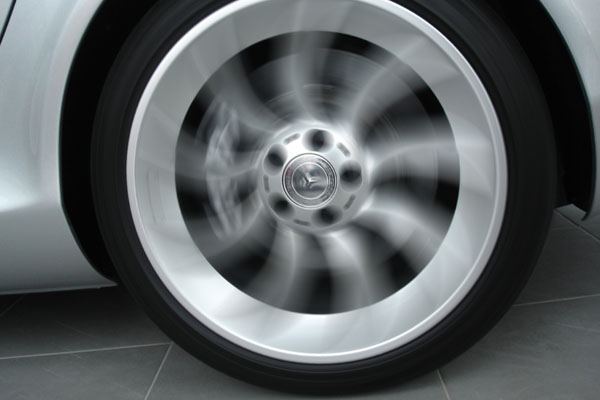# Ass­e­s­s­m­e­n­t 2: Ani­m­a­t­i­o­n with Code

For my first design, I was inspired by car wheels while i was walking home, the wheels were spinning so fast, it seems like their direction of spinning is random. I wanted to make an object that would resembles those properties.This picture is only an example of what I saw.

• Show Code
``````/* @pjs font=/uploads/16182/controllerthreeoblique.otf; */
/** @peep sketch */
float angle = 0.0;

void setup() {
size(200, 400);
background(0);
}

void draw() {
fill(0);
rect(0, 0, width, height);

textFont(font);
fill(255,255,0);
textAlign(CENTER,CENTER);
text("Design Lab", width/2, height/1.5);
stroke(255,255,0,10)

fill(255,255,0)
translate(width/2,height/2);
rotate(angle);
ellipse(0,0,100,20);
ellipse(20,0,100,20);
rect(40,40,10,10);
angle = angle + 5;
}``````

I abandoned this design after i realized it was too simple and that I was unable to come up with more things to add. For my next design, I was looking for mods to download for my game skyrim, and i was inspired by light rays while I was at http://www.nexusmods.com/skyrim/mods/30728/?.• Show Code
``````/* @pjs font=/uploads/16182/controllerone.otf; */
/**@peep sketch */
//declare variables
float x;
float y;
//setup
void setup() {
size(600, 400);
background(20);
x = random(width);
y = random(height);
frameRate(50);
}
void draw() {
if (frameCount % 9 == 0) {
rectMode(CORNER);
fill(0, 10);
rect(0, 0, width, height);
}
//3 random line (white and yellow lines) animation
x += random(-150,150);
y += random(-150,150);
x = constrain(x, 0, width);
y = constrain(y, 0, height);
stroke(255);
strokeWeight(4);
line(width/2,height/2,x*1.1,y);
stroke(255,255,0);
strokeWeight(4);
line(width/2,height/2,x,y);
//rectangle behind the text
stroke(255,255,0);
fill(0);
rectMode(CENTER);
rect(width/2,height/2.1,width*2,(width+height)/10);
//text
textFont(font);
fill(255,255,0);
textAlign(CENTER,CENTER);
text("Design Lab", width/2, height/2);
stroke(255,255,0,10)
}``````

My biggest weakness here is rescalability. My design can only rescale to a certain extend before becoming a mess.

I decided to add my first design into the new design. I devided "Design Lab" into two words to place the revolving wheel between them.

• Show Code
``````/* @pjs font=/uploads/16182/controllerone.otf; */
/**@peep sketch */
//declare variables
float x;
float y;
float angle = 0.0;
//setup
void setup() {
size(600, 400);
background(20);
x = random(width);
y = random(height);
frameRate(40);
}
void draw() {
if (frameCount % 9 == 0) {
noStroke();
rectMode(CORNER);
fill(0, 10);
rect(0, 0, width, height);
}
//3 random line (white and yellow lines) animation
x += random(-150,150);
y += random(-150,150);
x = constrain(x, 0, width);
y = constrain(y, 0, height);
stroke(255);
strokeWeight(4);
line(width/2,height/2,x*1.1,y);
stroke(255,255,0);
strokeWeight(4);
line(width/2,height/2,x,y);
//rectangle behind the text
stroke(255,255,0);
fill(0);
rectMode(CENTER);
rect(width/2,height/2.1,width*2,(width+height)/10);
//text
textFont(font);
fill(255,255,0);
textAlign(CENTER,CENTER);
text("Design", width/3.8, height/2);
text("Lab", width/1.3, height/2);
stroke(255,255,0,10)
//revolving wheel
noStroke();
fill(255);
translate(width/1.8,height/2.15);
rotate(angle);
ellipse(4,5,50,8);
angle = angle + 5;

}``````

Below with different dimensions:

• Show Code
``````/* @pjs font=/uploads/16182/controllerone.otf; */
/**@peep sketch */
//declare variables
float x;
float y;
float angle = 0.0;
//setup
void setup() {
size(250, 400);
background(20);
x = random(width);
y = random(height);
frameRate(40);
}
void draw() {
if (frameCount % 9 == 0) {
noStroke();
rectMode(CORNER);
fill(0, 10);
rect(0, 0, width, height);
}
//3 random line (white and yellow lines) animation
x += random(-150,150);
y += random(-150,150);
x = constrain(x, 0, width);
y = constrain(y, 0, height);
stroke(255);
strokeWeight(4);
line(width/2,height/2,x*1.1,y);
stroke(255,255,0);
strokeWeight(4);
line(width/2,height/2,x,y);
//rectangle behind the text
stroke(255,255,0);
fill(0);
rectMode(CENTER);
rect(width/2,height/2.1,width*2,(width+height)/10);
//text
textFont(font);
fill(255,255,0);
textAlign(CENTER,CENTER);
text("Design", width/3.8, height/2);
text("Lab", width/1.3, height/2);
stroke(255,255,0,10)
//revolving wheel
noStroke();
fill(255);
translate(width/1.8,height/2.15);
rotate(angle);
ellipse(4,5,50,8);
angle = angle + 5;

}``````
• Show Code
``````/* @pjs font=/uploads/16182/controllerone.otf; */
/**@peep sketch */
//declare variables
float x;
float y;
float angle = 0.0;
//setup
void setup() {
size(400, 200);
background(20);
x = random(width);
y = random(height);
frameRate(40);
}
void draw() {
if (frameCount % 9 == 0) {
noStroke();
rectMode(CORNER);
fill(0, 10);
rect(0, 0, width, height);
}
//3 random line (white and yellow lines) animation
x += random(-150,150);
y += random(-150,150);
x = constrain(x, 0, width);
y = constrain(y, 0, height);
stroke(255);
strokeWeight(4);
line(width/2,height/2,x*1.1,y);
stroke(255,255,0);
strokeWeight(4);
line(width/2,height/2,x,y);
//rectangle behind the text
stroke(255,255,0);
fill(0);
rectMode(CENTER);
rect(width/2,height/2.1,width*2,(width+height)/10);
//text
textFont(font);
fill(255,255,0);
textAlign(CENTER,CENTER);
text("Design", width/3.8, height/2);
text("Lab", width/1.3, height/2);
stroke(255,255,0,10)
//revolving wheel
noStroke();
fill(255);
translate(width/1.8,height/2.15);
rotate(angle);
ellipse(4,5,50,8);
angle = angle + 5;

}``````

As stated in the university website, the design lab is committed to interdisciplinary, design-led research that leads to improved interactions and experiences through and with digital technology.

The revolving wheel between "Design" and "Lab" represent continous efforts, while the light rays gives off positive energy. It also represent light rays from a light bulb which is the popular use to visualise "an idea". The logo is simple and yet both aesthetically and conceptually engaging. I believe my logo can represent the aim and the nature of Design Lab.